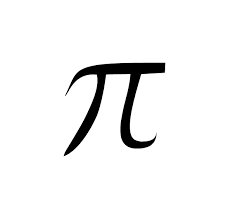# Pi - The most important but weird numberThe famous Indian astronomer, Aryabhata, approximated the value of $\pi$ as $3.1416$ and then expressed it as a continued fraction of the form

$\large a+\frac{1}{b+\frac{1}{c+\frac{1}{d}}},$

where $a,b,c,d$ are positive integers. Find $a+b+c+d$.


Details and Assumptions:

• Use the approximation $\pi = 3.1416$.
×